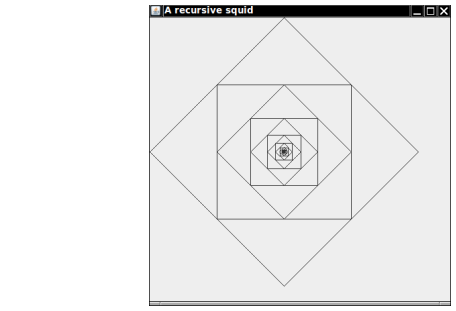A recursive “squid” is a square in a diamond containing another “squid”.

Your task is to implement the draw method of the squid. Draw the diamond, draw the square, then make another Squid with half the radius, and call its draw method. However, if the radius is too small, do nothing.

Viewer and component classes have been provided.

Here is a sample program output:Complete the following file:

### Squid.java

Use the following files:

SquidComponent.java

```import java.awt.Graphics;
import java.awt.Graphics2D;
import java.awt.geom.Point2D;
import javax.swing.JComponent;

public class SquidComponent extends JComponent
{
public void paintComponent(Graphics g)
{
Graphics2D g2 = (Graphics2D) g;
Squid s = new Squid(new Point2D.Double(200, 200), 200);
s.draw(g2);
}
}
```

SquidViewer.java

```import javax.swing.*;

public class SquidViewer
{
public static void main(String[] args)
{
JFrame frame = new JFrame();

frame.setSize(450, 450);
frame.setTitle("A recursive squid");
frame.setDefaultCloseOperation(JFrame.EXIT_ON_CLOSE);

SquidComponent component = new SquidComponent();